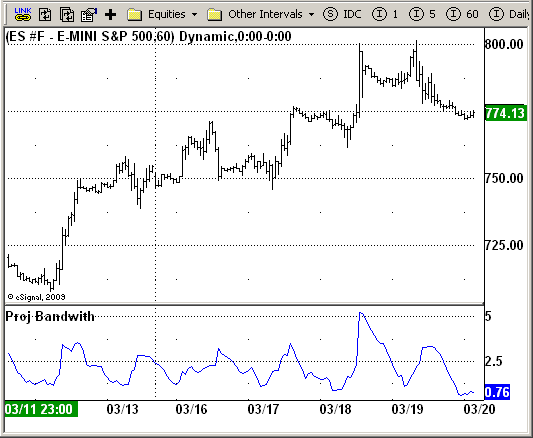# Proj Bandwith

ICE Data Services -

ProjBandwith.efs
EFSLibrary - Discussion Board

File Name: ProjBandwith.efs

Description:
This Indicator plots the Projected Bandwidth

Formula Parameters:

• Length : 14

Notes:

Projection bands, a new method using trading bands, projects market data forward along the data trend with the maxima and minima of the projections defining the band. The method provides another means of signaling potential changes for market direction relative to the trend.

This is an useful oscillator, the third component of the new method, the projection bandwidth indicator which is computed as the width compared with the average of the bands expressed as a percentage:

`PB = 200 x (PU - PL)/(PU + PL)`

ProjBandwith.efsEFS Code:

```/*********************************
Provided By:
eSignal (Copyright c eSignal), a division of Interactive Data
Formula Script (EFS) is for educational purposes only and may be
modified and saved under a new file name.  eSignal is not responsible
for the functionality once modified.  eSignal reserves the right
to modify and overwrite this EFS file with each new release.

Description:
This Indicator plots the Projected Bandwidth

Version:            1.0  03/18/2009

Formula Parameters:                     Default:
Length                              14

Notes:
Projection bands, a new method using trading bands, projects market
data forward along the data trend with the maxima and minima of the
projections defining the band. The method provides another means of
signaling potential changes for market direction relative to the trend.

This is an useful oscillator, the third component of the new method, the
projection bandwidth indicator which is computed as the width compared
with the average of the bands expressed as a percentage:
PB = 200 x (PU - PL)/(PU + PL)

**********************************/

var fpArray = new Array();
var bInit = false;

function preMain() {
setPriceStudy(false);
setShowTitleParameters(false);
setStudyTitle("Proj Bandwith");
setCursorLabelName("PB");
var x=0;
fpArray[x] = new FunctionParameter("Length", FunctionParameter.NUMBER);
with(fpArray[x++]){
setLowerLimit(1);
setDefault(14);
}
}

var xPB = null;

function main(Length) {
var nState = getBarState();
var nPB = 0;
if (nState == BARSTATE_ALLBARS) {
if (Length == null) Length = 14;
}
if (bInit == false) {
xPB = efsInternal("Calc_PB", Length);
bInit = true;
}
nPB = xPB.getValue(0);
if (getCurrentBarCount() < Length || nPB == null) return;
return nPB;
}

function Calc_PB(Length) {
var SL = 0;
var SH = 0;
var SumBars = Length * (Length - 1) * 0.5;
var SumSqrBars = (Length - 1) * Length * (2 * Length - 1) / 6;
var Sum1_1 = 0;
var Sum1_2 = 0;
var SumY1 = 0;
var SumY2 = 0;
var i = 0;
for (i = 0; i < Length; i++)  {
Sum1_1 += i * low(-i);
Sum1_2 += i * high(-i);
SumY1 += low(-i);
SumY2 += high(-i);
}
var Sum2_1 = SumBars * SumY1;
var Sum2_2 = SumBars * SumY2;
var Num1_1 = Length * Sum1_1 - Sum2_1;
var Num1_2 = Length * Sum1_2 - Sum2_2;
var Num2 = SumBars * SumBars - Length * SumSqrBars;
SL = Num1_1 / Num2;
SH = Num1_2 / Num2;
var Value2 = 0;
var Value3 = 0;
var PL = 0;
var PU = 0;
for (i = 1; i <= Length; i++) {
Value2=low(-i + 1) + (SL * i - 1);
Value3=high(-i + 1) + (SH * i - 1);
if (i == 1) {
PL=Value2;
PU=Value3;
};
if (Value2 < PL) PL = Value2;
if (Value3 > PU) PU = Value3;
}
var PB=200 * (PU - PL) / (PU + PL);
return PB;
}```